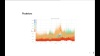webinar

# Bayesian Covariance for Portfolio OptimizationUncertainty quantified as probability is the rock upon which Bayesian inference is built. The instability of sample covariance matrices leads to major problems in Markowitz portfolio optimization. Max Margenot, Academia and Data Science Lead at Quantopian, uses probabilistic programming to compute probability distributions on the covariance of a set of assets. This yields a more robust estimate of their variation and factors uncertainty into how we calculate weights for a portfolio of assets.

You can find the slides here.